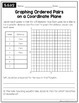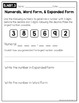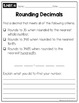# Open-Ended Math Pages - 5th GradeSubject
Resource Type
File Type

PDF

(3 MB|26 pages)
Standards
Also included in:
1. This BUNDLE includes everything you need for 5th grade math!!★ Interactive Notebook Pages★ Math Quick Checks ★ Math Menus (Perfect for homework or early finishers!!)★ Open-Ended Math Pages★ Math Exit Slips★ Math Mastery Task Cards★ Printables for DecimalsFeel free to email with any questions or comm
\$72.00
\$57.50
Save \$14.50
• Product Description
• Standards
This resource was designed to encourage students go beyond basic computation and take their understanding of the common core standards to the next level. Often times students are asked to write about how they solve a problem, come up with problems of their own, and demonstrate their understanding in a variety of ways.

These math pages give students the opportunity to explain their thinking, create their own word problems, make observations, make lists, draw conclusions, and much more.

These would work great for math centers, group work, or as assessments.

This product includes 26 math pages covering all of the Common Core Math Standards for 5th Grade...

Parentheses, Brackets, & Braces (5.OA.1)
Interpret Numerical Expressions (5.OA.2)
Numerical Patterns on the Coordinate Plane (5.OA.3)
Place Value Understanding (5.NBT.1)
Powers of 10 (5.NBT.2)
Numerals, Word Form & Expanded Form (5.NBT.3)
Comparing Decimals (5.NBT.3)
Rounding Decimals (5.NBT.4)
Multiplication (5.NBT.5)
Dividing Whole Numbers (5.NBT.6)
Multiplying & Dividing Decimals (5.NBT.7)
Adding & Subtracting Mixed Number (5.NF.1)
Word Problems: Adding & Subtracting Fractions (5.NF.2)
Relating Fractions & Division (5.NF.3)
Multiplying Fractions (5.NF.4/6)
Understanding Multiplication of Fractions & Whole Numbers (5.NF.5)
Dividing with Fractions (5.NF.7)
Measurement Conversions (5.MD.1)
Line Plots (5.MD.2)
Understanding & Measuring Volume (5.MD.3/4)
Volume of Rectangular Prisms (5.MD.5)
Graphing Ordered Pairs on a Coordinate Plane (5.G.1/2)
Classifying Two-Dimensional Figures (5.G.3)
Hierarchy of Two-Dimensional Figures (5.G.4)

Please check out my extensive preview to see sample pages.

Please e-mail me if you have any questions at CreateTeachShare@gmail.com

**PLEASE NOTE: DUE TO THE OPEN-ENDED NATURE OF THESE PAGES, AN ANSWER KEY IS ONLY PROVIDED FOR CERTAIN PAGES.

Check out all of my 5th Grade Interactive Math Notebooks, Worksheets, & Activities:
5th Grade Interactive Math Notebook: OA & NBT
5th Grade Interactive Math Notebook: NF
5th Grade Interactive Math Notebook: MD & G
Common Core Math Worksheets (for all 5th grade standards)
5th Grade Interactive Math Notebook {BUNDLE}
Classify two-dimensional figures in a hierarchy based on properties.
Understand that attributes belonging to a category of two-dimensional figures also belong to all subcategories of that category. For example, all rectangles have four right angles and squares are rectangles, so all squares have four right angles.
Represent real world and mathematical problems by graphing points in the first quadrant of the coordinate plane, and interpret coordinate values of points in the context of the situation.
Use a pair of perpendicular number lines, called axes, to define a coordinate system, with the intersection of the lines (the origin) arranged to coincide with the 0 on each line and a given point in the plane located by using an ordered pair of numbers, called its coordinates. Understand that the first number indicates how far to travel from the origin in the direction of one axis, and the second number indicates how far to travel in the direction of the second axis, with the convention that the names of the two axes and the coordinates correspond (e.g., 𝘹-axis and 𝘹-coordinate, 𝘺-axis and 𝘺-coordinate).
Solve real world problems involving division of unit fractions by non-zero whole numbers and division of whole numbers by unit fractions, e.g., by using visual fraction models and equations to represent the problem. For example, how much chocolate will each person get if 3 people share 1/2 lb of chocolate equally? How many 1/3-cup servings are in 2 cups of raisins?
Total Pages
26 pages
Included
Teaching Duration
1 Year
Report this Resource to TpT
Reported resources will be reviewed by our team. Report this resource to let us know if this resource violates TpT’s content guidelines.